[ITOC-ch4] DECIDABILITY

Recall:

Recognizer

• if $w∈L$, than M must accept w.
• if $w∉L$, than M may loop when input w.

Decider

• if $w∈L$, than M must accept w.
• if $w∉L$, than M must reject w.

4.1 DECIDABLE LANGUAGES

DECIDABLE PROBLEMS CONCERNING REGULAR LANGUAGES

$A_{DFA} = \{ \ <B,w> \ | \ B \ is \ a \ DFA \ that \ accepts \ input \ string \ w \}$.

Proof that it’s a decidable language by designing a decider

• M = “On input <B, w>, where B is a DFA and w is a string:
• Simulate B on input w.
• If the simulation ends in an accept state, accept . If it ends in a nonaccepting state, reject .”

$A_{NFA} = \{ \ <B, w> \ | \ B \ is \ an \ NFA \ that \ accepts \ input \ string \ w \}$.

Proof

• Convert NFA to DFA

$A_{REX} = \{ \ <R, w> \ | \ R \ is \ a \ regular \ expression \ that \ generates \ string \ w \}$.

Proof

• Convert RE to NFA

$E_{DFA} = \{ \ < A > | \ A \ is \ a \ DFA \ and \ L(A) = ∅ \}$.

Proof

• Mark all states reachable from start state. accept if no accept state is marked

$EQ_{DFA} = \{ \ <A, B> \ | \ A \ and \ B \ are \ DFA \ s \ and \ L(A) = L(B) \}$.

Proof

• Construct L( C ) = L( A ) xor L( B ), test if L( C ) $\in E_{DFA}$

DECIDABLE PROBLEMS CONCERNING CONTEXT-FREE LANGUAGES

$A_{CFG} = \{ \ <G, w> \ | \ G \ is \ a \ CFG \ that \ generates \ string \ w \}$

if G were in Chomsky normal form, any derivation of w has 2n − 1 steps, where n is the length of w.

Proof

• List all derivations with 2n − 1 steps, check if w is in them

$E_{CFG} = \{ \ < G > | \ G \ is \ a \ CFG \ and \ L(G) = ∅ \}$.

Proof

• Mark all terminal symbols and Mark all variables that can derive into marked symbols

$EQ_{CFG} = \{ \ <G, H> \ | \ G \ and \ H \ are \ CFG \ s \ and \ L(G) = L(H) \}$.

Not decidable (P226)

Every context-free language is decidable.

Proof:

• Use TM S that decide $A_{CFG}$ ( if w is generated by G )

• Let G be a CFG for the CFL A we want to decide

• Run S on <G,w>, if accept, means that $w \in G$ therefore $w \in A$, accept

4.2 UNDECIDABILITY

$A_{TM} = \{ \ <M, w> \ | \ M \ is \ a \ TM \ and \ M \ accepts \ w \}$.

THE DIAGONALIZATION METHOD

same size

• Set A and set B are the same size if there is a correspondence f : A→B.

countable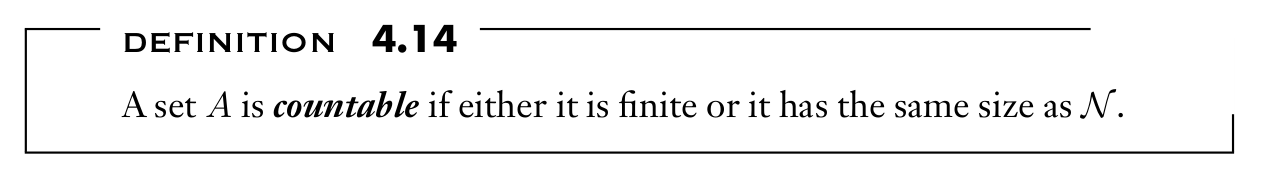Ex: $Q = \{ \frac{m}{n} | m, n ∈ N \}$ is countable

uncountable

Ex: the set of real numbers $R$ is uncountable

using diagonalization method

ensure that $x \neq f (n)$ for any n, therefore the correspondence to $N$ failed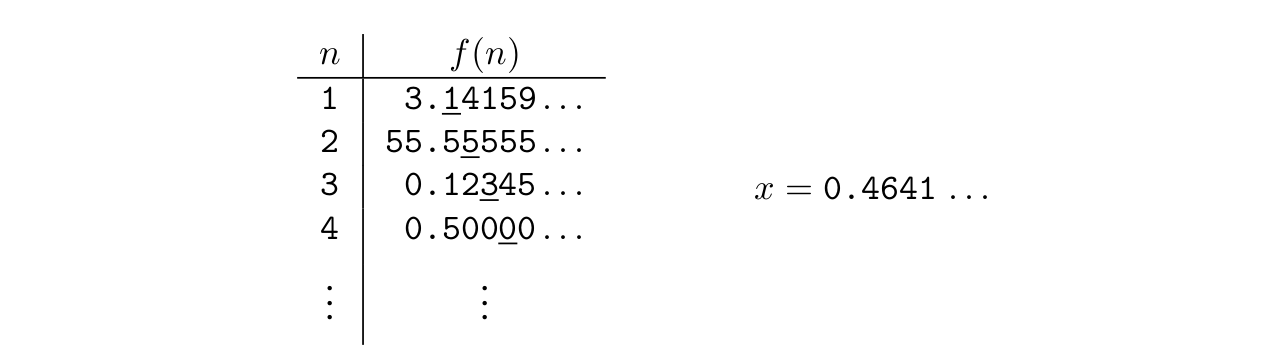Some languages are not Turing-recognizable.

Proof

• Set of all Turing machines $M$ is countable

• (图灵机的个数 == 图灵机识别的语言的个数 == 自然数的个数)

• each TM M has an encoding into a string \

• and set of all strings $Σ^ ∗$is countable ( set of finite length strings )
• Set of all languages $L$ is uncountable

• (所有语言的个数 == 实数的个数)

• $B$, the set of all infinite length binary sequence, is uncountable as $R$

• $L$, the set of all languages over alphabet $Σ$, have a correspondence with $B$
• Each language $A ∈ L$ has a unique sequence in $B$.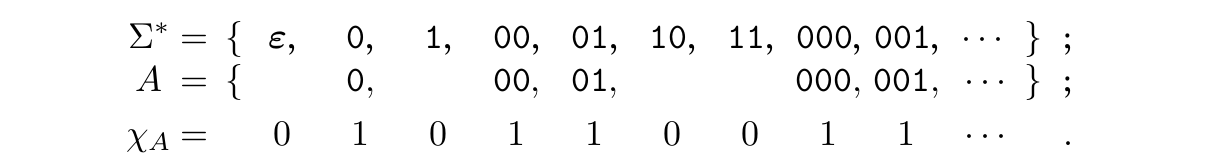• therefore $L$ is larger than $L_{TM}$

AN UNDECIDABLE LANGUAGE

$A_{TM} = \{ \ <M, w> \ | \ M \ is \ a \ TM \ and \ M \ accepts \ w \}$.

$A_{TM}$ is Turing-recognizable

• Simulate M on w
• If M ever enters its accept state, accept ; if M ever enters its reject state, reject ; If M loops, loop.

$A_{TM}$ is undecidable

Proof

• Assume we have the decider $H$ to decide whether M accept w• construct a new TM $D$ with $H$ as a subroutine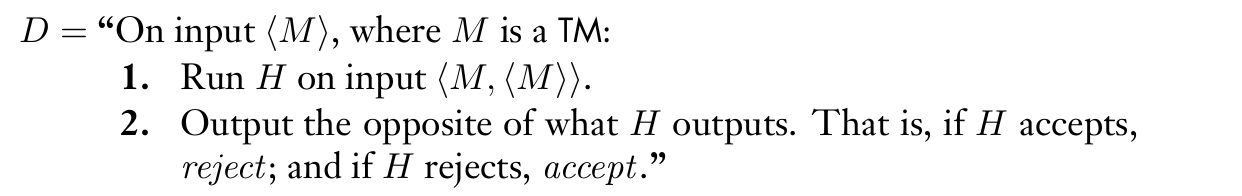• Contradiction when we run D with its own description \ as inputdiagnolization in the proof

• first list the table of whether $M_i$ accepts $<M_j>$
• construct $H$ so that $H$ reject when $M_i$ doesn’t accept $<M_j>$
• Finally the contradiction in $D$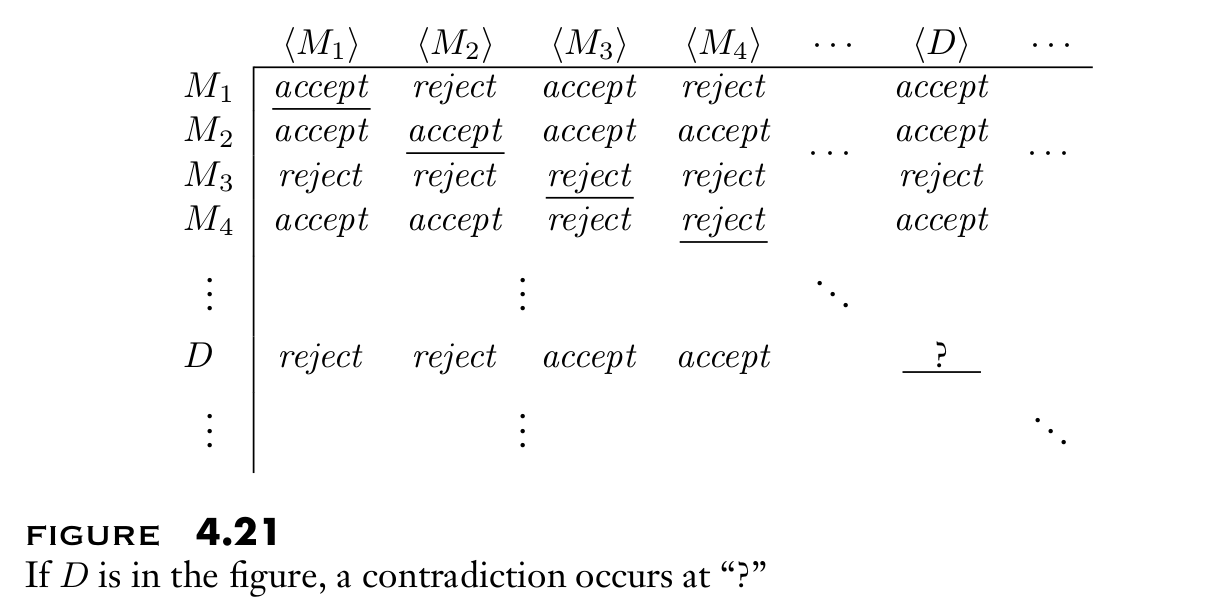A TURING-UNRECOGNIZABLE LANGUAGE

A language is decidable iff it is Turing-recognizable and co-Turing recognizable.

Proof

• if $A$ decidable, $\bar A$ is decidable, therefore both are recognizable

• if both recognizable, we can construct decider for $A$, therefore $A$ is decidable

Run their recognizer $M_1$ and $M_2$ simultaneously. If $M_1$ accepts, accept ; if $M_2$ accepts, reject.

$\overline { A_{TM} }$ is not Turing-recognizable.